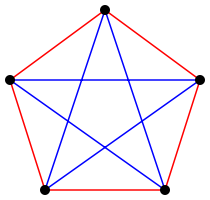# An Upper Bound on Ramsey Numbers (Revision Season)

First published May 02, 2019

Tags:

I will present a short argument on an upper bound for $$r(s)$$, the Ramsey Number associated with the natural number $$s$$.

The reader need only know some basic graph theory but any familiarity with Ramsey theory may help the reader appreciate the result more.

## 1. Preliminaries

Definition 1. (Ramsey Numbers) The Ramsey Number associated with the natural number $$s$$, denoted $$r(s)$$, is the least such $$n \in \mathbb{N}$$ such that whenever the edges of the complete graph on $$n$$ vertices (denoted $$K_n$$) are coloured with two colours, there must exists a monochromatic $$K_s$$ as a subgraph.

Definition 2. (Off-diagonal Ramsey Numbers) Let $$r(s,t)$$ be the least $$n \in \mathbb{N}$$ such that whenever the edges of $$K_n$$ are 2-coloured (say, red and blue), we have that there must exist either a red $$K_s$$ or a blue $$K_t$$ as a subgraph.

Some immediate properties that follow from the definitions are that for all $$s,t \in \mathbb{N}$$, we have

$r(s,s) = r(s), \\ r(s,2) = s,$

and

$r(s,t) = r(t,s).$

If you’re struggling to see why the second one is true, recall that the complete graph on 2 vertices is just a single edge. One may question whether these numbers necessarily exist. This is known as Ramsey’s theorem, which I will now state conveniently without proof.

Theorem 1. (Ramsey’s Theorem) $$r(s,t)$$ exists for all $$s,t \geq 2$$, and we have that

$r(s,t) \leq r(s-1,t) + r(s,t-1)$

for all $$s,t \geq 3$$.

To help illustrate what we are talking about, consider this colouring of the complete graph on 5 vertices.Inspection reveals that there is no completely red (or blue) triangle, which in fact constitutes a proof by counter example that $$r(3) > 5$$. In fact, it can be shown that $$r(3) = 6$$, that is, there does not exist a 2-colouring of $$K_6$$ that contains no monochromatic triangles.

## 2. An Upper Bound

Ramsey Numbers are notoriously difficult to calculate exactly - it is almost exclusively done by proving tighter and tighter bounds on them until we have equality of some description. I will prove one of these aforementioned bounds.

Lemma 2. We have that for any $$s,t \geq 2$$,

$r(s,t) \leq { {s+t-2}\choose{s-1} }.$

Proof. This result is shown by induction on $$s+t$$ using the inequality in Theorem 1. As a base case, suppose that either $$s$$ or $$t$$ equals $$2$$. Without loss of generality, due to the symmetric nature of $$r(s,t)$$, suppose $$s = 2$$. We have that

$r(2,t) = t = { {t}\choose{1} } = { {s + t - 2}\choose{s-1} }$

and we are done. Now suppose that $$s,t \geq 3$$. Then by Theorem 1 and Pascal’s identity, we have

\begin{align} r(s,t) & \leq r(s-1,t) + r(s,t-1) \\ & \leq { {s + t - 3}\choose{s-2} } + { {s + t - 3}\choose{s-1} } \\ & = { {s + t - 2}\choose{s-1} } \end{align}

And the induction is complete. //

Next we shall prove an inequality regarding the binomial coefficient itself.

Lemma 3. For any $$m \in \mathbb{N}$$, we have that

${ {2m}\choose{m} } < \frac{2^{2m}}{\sqrt{2m+1}}.$

Proof. We proceed by induction. When $$m=1$$ we have that

${ {2}\choose{1} } = 2 < \frac{4}{\sqrt{3}}.$

Let $$m \geq 1$$. We have

\begin{align} \frac{1}{2^{2(m+1)}} { {2(m+1)}\choose{m+1} } & = \frac{(2m+2)!}{2^{2m+2}((m+1)!)^2} \\ & = \frac{(2m)!(2m+1)(2m+2)}{2^{2m} (m!)^2 (m+1)^2} \\ & = \frac{(2m)!}{2^{2m} (m!)^2}\frac{2m+1}{2m+2} \\ & < \frac{1}{\sqrt{2m+1}} \frac{2m+1}{2m+2} \\ & = \frac{\sqrt{2m+1}}{2m+2}. \end{align}

It remains to be shown that $$\frac{\sqrt{2m+1}}{2m+2} \leq \frac{1}{\sqrt{2m+3}}$$. We do this by considering squares of both sides. Observe that

$(\sqrt{2m+1} \sqrt{2m+3})^2 = 4m^2 + 8m + 3 < 4m^2 + 8m + 4 = (2m+2)^2$

and from this our result follows. //

We now tie these Lemmas together to give our main result.

Theorem 4. For any $$s\geq 2$$, we have that

$r(s) \leq \frac{4^s}{\sqrt{s}}.$

Proof. In this proof we use a slightly weaker version of Lemma 3, namely that

${ {2m}\choose{m} } \leq \frac{2^{2m}}{\sqrt{2m}}.$

First, we simply recall that $$r(s) = r(s,s)$$, so by Lemma 2 we have

$r(s) \leq { {2s-2}\choose{s-1} }.$

Next, using (the slightly weakened) Lemma 3 and some algebra we see

\begin{align} { {2s-2}\choose{s-1} } & \leq \frac{2^{2s-2}}{\sqrt{2s-2}} \\ & = \frac{4^s 2^{-2}}{\sqrt{2s-2}} \\ & \leq \frac{4^s}{\sqrt{2} \sqrt{s-1}}. \end{align}

It remains to be shown that $$\sqrt{2} \sqrt{s-1} \geq \sqrt{s}$$. Observe that for $$s\geq 2$$

$\sqrt{\frac{1}{2}} \leq \sqrt{1-\frac{1}{s}} = \sqrt{\frac{s-1}{s}}$

from which our result follows.//

## 3. Closing Remarks

This problem was found in a problem sheet from my Combinatorics course, and I wrote this up as a short mathematical writing exercise and for a revision break. I plan to write up more posts on interesting questions posed in problem sheets or elsewhere as a way to keep me sane in the next month or so. (Edit, 6/6/19: I did not manage to write about anything else.)

It is interesting to note that proving the stronger version of Lemma 3 presented ended up being much easier than showing the weaker version used in Theorem 4. A big thanks to the people on the Mathematics Stack Exchange who helped me out with this problem and in particular Lemma 3. See the question and answers here.